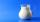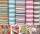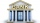Homework

In the crate are 18 plums, 27 apricot and 36 nuts. How many pieces of fruit left in the crate when Peter took 8 ninth:

1. nuts
2. apricots
3. fruit
4. drupe

Result

pcs of fruit:  45
pcs of fruit:  21
pcs of fruit:  5
pcs of fruit:  5

Solution:Leave us a comment of this math problem and its solution (i.e. if it is still somewhat unclear...):Be the first to comment!To solve this verbal math problem are needed these knowledge from mathematics:

Need help calculate sum, simplify or multiply fractions? Try our fraction calculator.

Next similar math problems:

1. PearsThere were pears in the basket, I took two-fifths of them, and left six in the basket. How many pears did I take?
2. Passenger boatTwo-fifths of the passengers in the passenger boat were boys. 1/3 of them were girls and the rest were adult. If there were 60 passengers in the boat, how many more boys than adult were there?
3. Tank of fuelA 14.5-gallon tank of fuel is 3/4 full. How many more gallons will it take to fill up the tank?
4. MilkAt the kindergarten, every child got 1/5 liter of milk in the morning and another 1/8 liter of milk in the afternoon. How many liters were consumed per day for 20 children?
5. PackageThe package was 23 meters of textile. The first day sold 12.3 meters. How many meters of textile remained in the package?
6. SpendingPeter spends 1/5 of his earnings on his rent and he saves 2/7. What fraction of his earnings is left?
7. Cupcakes 2Susi has 25 cupcakes. She gives 4/5. How much does she have left?
8. KingKing had four sons. First inherit 1/2, second 1/4 , third 1/5 of property. What part of the property was left to the last of the brothers?
9. To improper fractionChange mixed number to improper fraction a) 1 2/15 b) -2 15/17
10. FractionDetermine for what x fraction ?:
11. Fraction + eqSolve following simple equation with fractions: -5/6(8+5b) = 75 + 5/3b
12. Write 2Write 791 thousandths as fraction in expanded form.
13. Ratio v2Decrease in the ratio 12:16 number 13.2.
14. My fatherMy father has a big farm. 6/8 of it were planted with mango trees, 1/2 of the remainder are guava trees and the rest 10 trees are santol trees. What is the number of all trees?
15. RatioIncrease in the ratio 20:4 number 18.5.
16. Pizza 4Marcus ate half pizza on monday night. He than ate one third of the remaining pizza on Tuesday. Which of the following expressions show how much pizza marcus ate in total?
17. Unknown numberI think the number - its sixth is 3 smaller than its third.